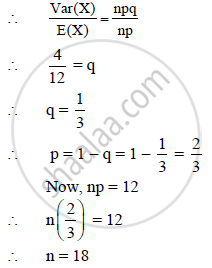Share

# If X ~ B (N, P) and E(X) = 12, Var(X) = 4, Then the Value of N Is__ - Mathematics and Statistics

#### Question

If X ~ B (n, p) and E(X) = 12, Var(X) = 4, then the value of n is _______

(A) 3

(B) 48

(C) 18

(D) 36

#### Solution

E(X) = np and Var (X) = npqIs there an error in this question or solution?

#### APPEARS IN

2012-2013 (October) (with solutions)
Question 4.1.3 | 2.00 marks

#### Video TutorialsVIEW ALL 

Solution If X ~ B (N, P) and E(X) = 12, Var(X) = 4, Then the Value of N Is__ Concept: Bernoulli Trials and Binomial Distribution - Calculation of Probabilities.
S